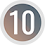# Surface Area of a Sphere## Surface Area of a Sphere

The total curved surface around the sphere is called the total surface area (TSA) of the sphere. Archimedes (6th century BC) discovered that the total surface area of a spherical object like tennis ball, football etc. is equal to the curved surface area of the circumscribing cylinder.

The figure given above is the cylinder circumscribing a sphere. Here diameter of the sphere AB is equal to the diameter PQ of the cylinder and diameter of sphere is equal to the height QR of cylinder.

We know that the curved surface area (CSA) of a cylinder = 2πrh

Now, by Archimedes principle on TSA of sphere,

Total surface area of sphere = curved surface area of the circumscribing cylinder
= 2πrh
= 2πr × d      [ h = d]
= 2πr × 2r    [ d = 2r]
= 4πr2
Total surface area of a sphere = 4πr2

### Surface area of a hemisphere

Hemisphere is one of the half part of a sphere when it is divided into two equal parts. It has two surfaces, one circular face (great circle) and another curved surface.
The radius of hemisphere is equal to the radius of the sphere. Since hemisphere is half part of sphere,

Curved surface area of hemisphere = ½ of curved surface area of sphere
= ½ × 4πr2
= 2πr2

Total surface area of hemisphere = CSA of hemisphere + area of circular surface
= 2πr2 + πr2
= 3πr2
TSA of hemisphere = 3πr2

### Workout Examples

Example 1: Find the total surface area of a sphere of diameter 21cm.

Solution: Here,
Diameter of sphere (d) = 21cm
radius of sphere (r) = 21/2 = 10.5cm

Now,
TSA of sphere = 4πr2
= 4 × 22/7 × (10.5)2
= 88/7 × 110.25
= 1386cm2

Thus, the total surface area of sphere is 1386cm2.

Example 2: Find the total surface area of a sphere if the circumference of the great circle is π cm.

Solution: Here,
Circumference of great circle = π cm
i.e.     2πr = π
or,     r = π/2π
or,     r = ½
or,     r = 0.5cm

Now,
TSA of sphere = 4πr2
= 4 × π × (0.5)2
= 4 × π × 0.25
= π cm2

Thus, the total surface area of sphere is π cm2.

Example 3: Find the total surface area of two hemispheres when a sphere of radius 7cm is cut into two halves.

Solution: Here,

Now,
The total surface area (TSA) of a hemisphere = 3πr2
= 2 × 22/7 × 72
= 2 × 22 × 7
= 308cm2

Total surface area of two hemisphere = 2 × 308cm2
= 616 cm2

Thus, the total surface area of two hemisphere is 616 cm2.

You can comment your questions or problems regarding the TSA of sphere and hemisphere here.

1.1.